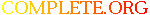Complete.Org: Mailing Lists: Archives: freeciv-dev: March 2002: [Freeciv-Dev] [RFC] approximations for win_chance# [Freeciv-Dev] [RFC] approximations for win_chance

[Top] [All Lists]

 To: freeciv-dev@xxxxxxxxxxx Subject: [Freeciv-Dev] [RFC] approximations for win_chance From: Gregory Berkolaiko Date: Sat, 23 Mar 2002 20:30:33 +0000 (GMT)

```Since freeciv-dev seems to be dead (or everyone is talking behind my
back), I'll continue flooding it with my spammy messages.

Although Raimar doesn't think that win_chance computation is heavy, I was
still thinking how we can approximate it's result without summing the
series.

The approximation used widely in the AI code (through kill_desire
equation) is
prob_to_win =
(HP_A * P_A * FP_A)^2 / ((HP_D * P_D * FP_D)^2 + (HP_A * P_A * FP_A)^2)
where
A stands for attacker, D stands for defender,
HP is hitpoints
P is the corresponding power (attack or defense)
FP is the firepower

I suspect that sometimes the values are not squared.

Recently I encountered an example where this approximation gives a wrong
Attacker = Stealth Bomber(HP=20, A=21, FP=2, cost=160)
Defender = Partisan fortified on a mountain with a river
(HP=20, D=27, FP=1, cost=50)

The exact answer (win_chance) is: .8845473776
The approximation (with squares) is: .7075812274
Approximation without squares is: .6086956522

0.177 absolute error might seem to be insignificant but the effect is that
the bomber doesn't want to risk trying to kill the partisans:
kill_desire (win_chance) is: 25 shields
kill_desire (approx w/squares) is: -11 shields

Recently I played a little bit with Maple and discovered that win_chance
can be very well approximated by
(HP_A * P_A * FP_A)^5 / ((HP_D * P_D * FP_D)^5 + (HP_A * P_A * FP_A)^5)

I don't know what is the meaning of the magic number 5, but it seems to
work: I plotted numerous graphs (*.mws is available upon request) and I
very rarely saw the absolute error be above 0.02.

In the example above my approximation gives: .9010694067 which is
0.0165 greater than the exact value.  This results in kill_desire being
29 shields.  I think it's not too bad.

In future I will probably base my computations on the exact win_chace,
to avoid stupid behaviour.  And when the unbelievers will see the time
consumed by win_chance soaring, we can convert to my approximation.  By
the way, it is really easy to cache the result of my approximation: there
are only two real parameters.

Best,
G.

```